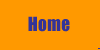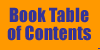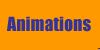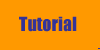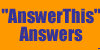"Answer This": Questions and Answers Fundamentals of Sound contains questions throughout labeled "Answer This," which are meant to stimulate active learning by the reader. To use these questions you should read them within the context of the book section in which they appear, attempt to formulate an answer, and then use this section only to check the correctness of your own answer. These questions are not numbered in the book and we refer to them by section number. To go to the question and answer, click on the Section number below. Some of the Answer-This questions also appear in the Tutorial section of this web site. Chapter 1 Sec. 1-C.  Wave motion versus medium motion. Sec. 1-D.  Wave velocity. Sec. 1-H.  What can you change? Sec. 1-I.   Pressure. Sec. 1-J.  Sounds through a wall. Chapter 2 Sec. 2-C.  Standing wave on a string fixed at both ends. Sec. 2-C.  Standing wave: Which mode? What frequency? Sec. 2-C.  Standing wave: What can you change? Chapter 3 Sec. 3-B.  Boundary conditions in a tube of air. Sec. 3-D.  Pressure and displacement wave graphs. Chapter 4 Chap 4 .  Resonance. Chapter 5 Sec. 5-A.  Intensity versus distance. Chapter 6 Sec. 6-A.  Phase. Sec. 6-C.  Graphical sum of two sinusoidal waves. Sec. 6-F.  Repeat time of a complex wave. Chapter 7 Sec. 7-C.  Square wave shapes. Sec. 7-D.  Repeat time of a complex wave. Sec. 7-E.  Repeat time of a filtered complex wave. Sec. 7-F.  Frequency spectrum. There are no Answer-This questions in Chapters 8 and 9. Chapter 10 Sec. 10-A.  When is work being done? Sec. 10-A.  Energy of a diver. Sec. 10-A.  Types of energy. Sec. 10-G.  Intensity and amplitude. Chapter 11 Sec. 11-G.  Logarithms. Sec. 11-H.  Decibels. Sec. 11-I.   Phons. Sec. 11-J.  Combined sound sources. Chapter 12 Sec. 12-B.  Lever. Sec. 12-B.  Trumpet bell.© William J. Mullin